## 8  The quantifiers

Having used the symbols ∃ and ∀, we are obliged to justify them in terms of the rules of natural deduction, or at least their categorical interpretation [Tay99, §§9.3–4] as we don’t want to get too heavily involved in syntax. In particular, the ability to substitute under a quantifier is another consequence of insisting that the adjunctions be internal.

[The letter E is used in the following two results to suggest an existential quantifier, not a nucleus.]

Proposition 8.1 If Σ!:Σ→ΣX has a left (or right) adjoint then Σp1Y→ΣX× Y also has one, and this automatically satisfies the Beck–Chevalley condition, the pullbacks in question being given by product projections as shown on the left below.Proof    If E⊣Σ! then FEY⊣Σp1 by Lemma 3.6.

Explicitly, for ω∈ΣX× Y,  put F(ω)≡ λ y.Ex.ω(x,y)); then for ψ∈ΣY,

 F(Σ p1(ψ))= λ y.E(λ x.ψ y)=λ y.EΣ!(ψ y) ⊑λ y. ψ y = ψ,

whilst ω(x,y)⇒Σ! Ex′.ω(x′,y)) ⇔ F p1ω)(y).

The Beck–Chevalley condition is naturality of E(−)X×(−)→Σ(−) with respect to f. Explicitly, ω↦λ z.Ex.ω(x, fz)) both ways round the square involving F and GEZ.         ▫

The preceding proof only required Σ to be exponentiating: the Euclidean principle comes into the next result.

Proposition 8.2 For X overt, every product projection p1:X× YY is pre-open, because

1. by the Euclidean principle, !:X1 is pre-open, i.e. ∃XX→Σ obeys the Frobenius law (cf. [JT84, Proposition V 3 1] for locales);
2. for any pre-open map f:XZ, the map f× Y:X× YZ× Y is also pre-open (cf. Bourbaki’s definition of proper maps, [Bou66, §10]).

Proof

1. For σ∈Σ and φ∈ΣX, let F(σ)≡ E(φ∧ Σ!(σ)):Definition 7.7 required E to be monotone, so F(σ)⇒ E!σ)⇒ σ. Then

 E(φ∧Σ!σ)⇔ F(σ)⇔ F(σ)∧ σ⇔  F(⊤)∧ σ⇔ E(φ)∧ σ

by the Euclidean principle.

2. Let φ∈ΣX× Z be a generalised element in the context Γ, so φ:Γ→ΣX× Z; then φ′≡λ x.φ(x,z) is a generalised element in the context Γ× Z, and this is how Definition 7.1 must be read. From Proposition 8.1, ∃f× Z φ=λ z.∃fx.φ(x,z)). Then for ψ∈ΣY× Z,
 ∃f× Z(φ∧Σf× Zψ) = λ z.∃f(λ x.φ(x,z)∧ ψ(f x,z)) = λ z.(∃f(λ x.φ(x,z))∧λ y.ψ(f x,z)) = ∃f× Z φ∧ ψ

▫

Proposition 8.3 By analogy with Proposition 6.11,

1. 1 is overt and compact.
2. If X and Y are both overt or both compact then so is X× Y.
3. If UX is an open subset of an overt object then U is itself an overt object.
4. Similarly for closed subsets of compact objects.

Proof    [a] ∃1≡ ∀1idΣ. [b] ∃X× Y≡ ∃X·∃YX≡ ∃Y·∃XY. [c,d] UX1 is a composite of open or proper maps.         ▫

[Beware that the letter I in the following two results denotes an “indexing object” not a Σ-splitting.]

Corollary 8.4 If the object I is overt then every algebra (A≡ ΣX) has internal I-indexed joins, ∧ distributes over them and they are preserved by homomorphisms.Dually, if I is compact then algebras have and homomorphisms preserve I-indexed meets.

Proof    These are re-statements of the Frobenius and Beck–Chevalley conditions.         ▫

Corollary 8.5

1. In classical topology, every object I is overt. Therefore algebras have all joins, and binary meet distributes over them, i.e. the algebras are frames, and the homomorphisms preserve joins. By the adjoint function theorem, Heyting implication exists in the algebras, and frame homomorphisms have (non-continuous) right adjoints.
2. In recursion, ℕ is overt, but other objects need not be. The algebras are sometimes called σ-frames.
3. ℕ is not compact, so ℕ-indexed meets need not be preserved.
4. If 0 and 2 are overt then each algebra is an internal distributive lattice, which we shall consider in the next section.         ▫

Now let’s think a bit about syntax, using [Tay99, §9.3].

Remark 8.6 Our category is a model of a fragment of predicate calculus in which each object names a (non-dependent) type, and contexts are products (cf. Theorem 4.2). Each open inclusion UX is a predicate x:X⊢φ(xprop, though we prefer to regard φ(x) as a generalised element of ΣX, rather than as a mono. Thus we interpret

 Γ⊢φ prop by φ∈Σ , i.e.   φ:Γ→Σ,  and Γ, φ1,φ2, …, φn⊢θ by φ1∧φ2∧⋯∧φn⇒θ∈Σ.

The effect of pullback Σp0p0* along a product projection p0:Γ× X→Γ is to add a variable x to the context (weakening, which is written x* in [Tay99]):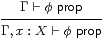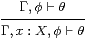If X is overt then this map Σp0 has a left adjoint ∃XX→Σ or ∃p0Γ× X→ΣΓ, which interprets existential quantification: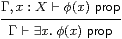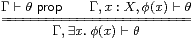The Beck–Chevalley condition is needed to ensure that, for any function f:Γ→Δ between types, the bijective correspondence on the right is preserved by Σf (substitution or cut along f), whilst the Frobenius law provides in a similar way for additional predicates that may be present in the context.

Remark 8.7 In Section 10 we shall encounter expressions of the forms

 ∃i· Σi · Σp0,      ∃p1·∃i· Σi · Σp1   and   ∃p1·∃i· Σi · Σp0

where i:RX× Y is the inclusion of an open binary relation classified by ρ:X× Y→Σ. Recall from Section 3 that ∃i· Σi ≡ρ∧(−), so it takes

 Γ, x:X,y:Y⊢ψ(x,y)     to     Γ, x:X,y:Y⊢ρ(x,y)∧ψ(x,y)

without changing the context. Hence the effect on Γ, x:X⊢φ(xprop of

 ∃i· Σi · Σp0 is Γ,x:X,y:Y⊢ρ(x,y)∧φ(x) prop ∃p1·∃i· Σi · Σp1 is Γ,y:Y⊢∃ x:X.ρ(x,y)∧φ(y) prop ∃p1·∃i· Σi · Σp0 is Γ,y:Y⊢∃ x:X.ρ(x,y)∧φ(x) prop

▫

This brief discussion of the rules of natural deduction and Corollary 10.11 about the direct image show logicians and categorists respectively that we are using the existential quantifier in the usual way. However, the dual of the Euclidean principle implies the dual Frobenius law for ∀X, which is something extra on top of the standard rules for the universal quantifier [Tay99, §9.4], namely that Σ!⊣∀X with the Beck–Chevalley condition.

Remark 8.8 Let φ∈ΣX be a decidable predicate on any overt compact object, so ∀ x.(φ(x)∨ψ(x)), where ψ≡ ¬φ∈ΣX. Then we have ∀ x.(φ(x)∨∃ y.ψ(y)), which is equivalent by the dual Frobenius law to (∀ x.φ(x))∨(∃ y.ψ(y)).

For decidable predicates on ℕ, this property is well known in recursion theory as the Markov principle [Mar47] [Ros86, §5.1].

For us it is not legitimate to write “∀ n:ℕ.φ(n)” because ℕ is not compact in topology or recursion (Remark 7.11). But consider its one-point compactification, ℕ; both ℕ and ℕ are (intended to be) overt, and the inclusion i:ℕ↪ℕ is dense in the sense that (∃ x:ℕ.ψ(x))⇔ (∃ n:ℕ.ψ(i n)). Although ℕ is not compact, if ⊤⇔ ∀ x:ℕ.φ(x)∈Σ then Σiφ=⊤∈Σ. Conversely, we may transfer Σ-predicates from ℕ to ℕ, but we cannot, unfortunately, do the same with decidable ones without prejudging the question for the extra point ∞∈ X.         ▫

We have a more encouraging result when we compare our universal quantifier for closed subsets with the standard one for all subsets in a topos. The following situation arises in synthetic domain theory (Remark 2.13), for the sheaves for a Lawvere–Tierney topology (Σ≡ Ωj in Example 2), and also for Ω↠Υ in Example 6.14 since this map is actually also mono.

[Eduardo Dubuc and Jacques Penon [DP86] called an object K of a topos compact if its universal quantifier ∀K satisfies the dual Frobenius law.]

Proposition 8.9 Suppose that Σ is an object that satisfies the Euclidean principle in a topos, so Σ classifies certain open subsets, whilst Ω classifies all subsets. If the object X is compact with respect to Σ then the interpretations of ∀X with respect to Σ and Ω agree, in the sense that the bottom face of the cube commutes: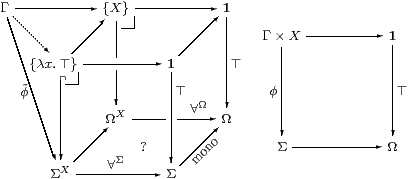Proof    To prove that the two routes ΣX→Ω are equal, it is enough to show that they both classify the Ω-subobject {λ x.⊤}⊂ΣX. The front and back faces of the cube are pullbacks by Proposition 7.10, as is the right face because Σ→Ω is mono by Remark 2.13. To show that the left face is also a pullback, consider any test Γ; the two routes Γ⇉ΩX are equal iff the square on the right commutes, which it does iff ∼φ=λ x.⊤, as required.         ▫

Remark 8.10 Of the other meanings that we might attribute to saying that the two notions of agree, one is trivially true in that ΣX≅ΣX, where X is the replete reflection of X, and another is trivially false in that every object is compact with respect to Ω, but not necessarily with respect to Σ.

Remark 8.11 It is not possible to adapt this argument to ∃ and ⊥ because, without excluded middle, characteristic maps with respect to ⊥:1→Ω in a topos need not be unique. An analogous result can nevertheless be achieved by imposing the open cover Lawvere–Tierney topology on the topos, but discussion of this relies on sheaf-theoretic methods, which are inappropriate for this paper.